GeeksforGeeks App
Open AppBrowser
Continue

# How to extract Time data from an Excel file column using Pandas?

Prerequisite: Regular Expressions in Python

In these articles, we will discuss how to extract Time data from an Excel file column using Pandas. Suppose our Excel file looks like below given image then we have to extract the Time from the Excel sheet column and store it into a new Dataframe column.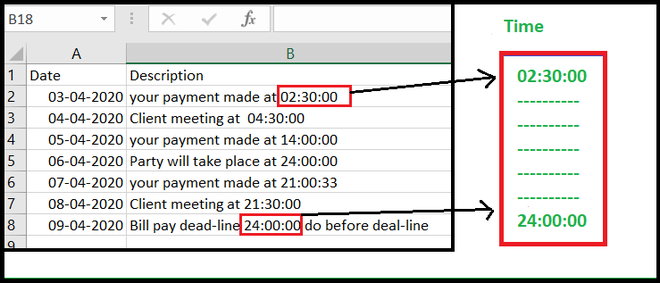Approach:

• Import the required module.
• Import data from Excel file.
• Make an extra column for store extracted time.
• Set Index for searching for extracting column.
• Define the pattern of Time format (HH: MM: SS).
• Search Time and assigning to the respective column in Dataframe.

Let’s see Step-By-Step-Implementation:

Step 1: Import the required module and read data from Excel file.

## Python3

 `# importing required module``import` `pandas as pd;``import` `re;`` ` `# Read excel file and store in to DataFrame``data ``=` `pd.read_excel(``"time_sample_data.xlsx"``);`` ` `print``(``"Original DataFrame"``)``data`

Output: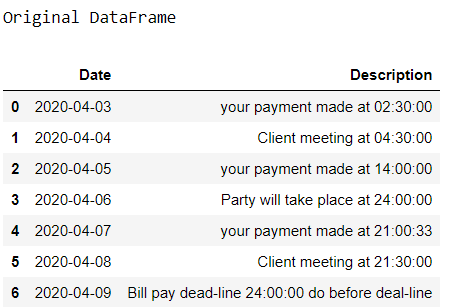Step 2: Make an extra column for storing Time data.

## Python3

 `# Create column for Time``data[``'New time'``] ``=` `None``data`

Output: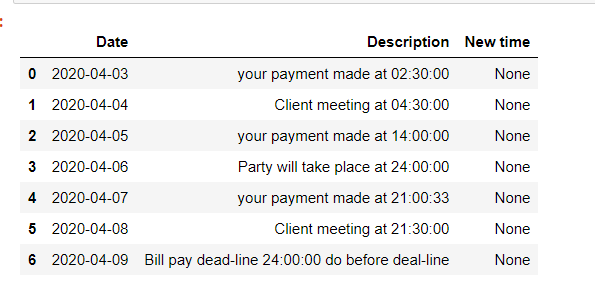Step 3: Set Index for searching

## Python3

 `# set index``index_set ``=` `data.columns.get_loc(``'Description'``)``index_time ``=` `data.columns.get_loc(``'New time'``)`` ` `print``(index_set, index_time)`

Output:

```1 2
```

Step 4: Defining the Regular expression (regex) for the time.

Regex for time HH/ MM/ SS format:

```[0-24]{2}\:[0-60]{2}\:[0-60]{2}.
```

## Python3

 `# define time pattern``time_pattern ``=` `r``'([0-24]{2}\:[0-60]{2}\:[0-60]{2})'`

Step 5: Search Time and assigning to the respective column in Dataframe.

For searching the time using regex in a string we are using re.search() function of re library.

## Python3

 `# searching the entire DataFrame``# with Time pattern``for` `row ``in` `range``(``0``, ``len``(data)):``   ` `    ``time ``=` `re.search(time_pattern,``                     ``data.iat[row,index_set]).group()``     ` `    ``data.iat[row, index_time] ``=` `time``     ` `print``(``"Final DataFrame"``)    ``data`

Output: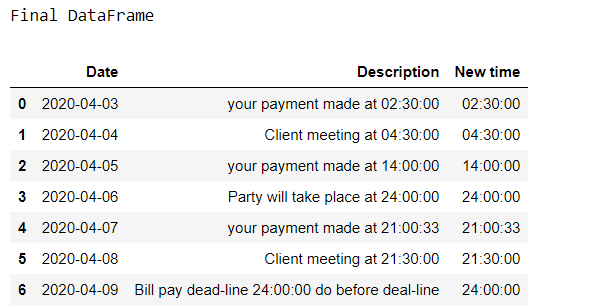Complete Code:

## Python3

 `# importing required module``import` `pandas as pd;``import` `re;`` ` `data ``=` `pd.read_excel(``"time_sample_data.xlsx"``);``print``(``"Original DataFrame"``)``print``(data)`` ` `# Create column for Date``data[``'New time'``]``=` `None``print``(data)`` ` `# set index``index_set``=` `data.columns.get_loc(``'Description'``)``index_time``=``data.columns.get_loc(``'New time'``)``print``(index_set,index_time)`` ` `# define the time pattern in HH:MM:SS``time_pattern``=` `r``'([0-24]{2}\:[0-60]{2}\:[0-60]{2})'`` ` `#searching dataframe with time pattern``for` `row ``in` `range``(``0``, ``len``(data)):``    ``time``=` `re.search(time_pattern,data.iat[row,index_set]).group()``    ``data.iat[row,index_time] ``=` `time``     ` `print``(``"\n Final DataFrame"``)    ``data`

Output: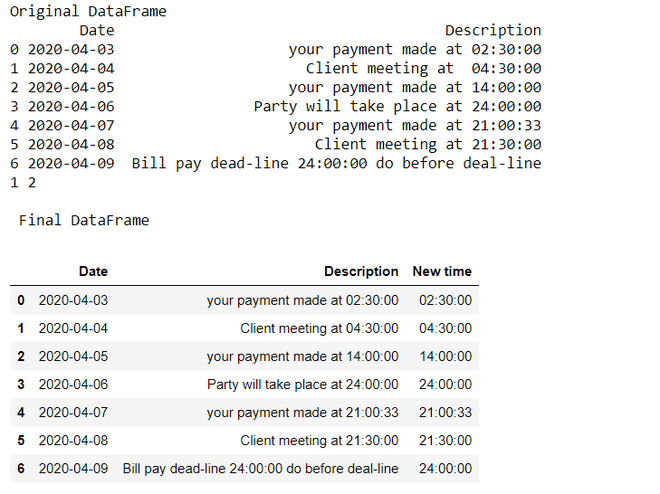Note: Before running this program, make sure you have already installed xlrd library in your Python environment.

My Personal Notes arrow_drop_up